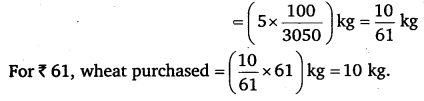# Class 6 Maths NCERT Solutions for Chapter 12 Ratio and Proportion Ex – 12.3

## Ratio and Proportion

Question 1.
If the cost of 7 m of cloth is X 294, find the cost of 5 m of cloth.

Solution:
Cost of 7 m of cloth = ₹ 294
∴ Cost of 1 m of cloth = ₹ (294 7)
= ₹ 42
∴ Cost of 5 m of cloth = ₹ (42 x 5)
= ₹ 210.Question 2.
Ekta earns X1500 in 10 days. How much will she earn in 30 days?

Solution:
Ekta’s earning of 10 days = ₹ 1500
Ekta’s earning of 1 day = ₹ (1500 ÷ 10)
= ₹ 150
∴ Ekta’s earning of 30 days = ₹ (150 x 30)
= ₹ 4500

Question 3.
If it has rained 276 mm in the last 3 days, how many cm of rain will fall in one full week (7 days) ? Assume that the rain continues to fall at the same rate.

Solution:
Rainfall in 3 days = 276 mm
∴ Rainfall in 1 day = (276 + 3) mm = 92 mm
Rainfall in 1 week (i.e., 7 days) = (92 x 7) mm
= 644 mm.
In cm, rainfall in one full week = 644 x$\frac { 1 }{ 10 }$ cm [1 m =$\frac { 1 }{ 10 }$ cm]
= 64.4 cm

Question 4.
Cost of 5 kg of wheat is ? 30.50.

(a) What will be the cost of 8 kg of wheat?
(b) What quantity of wheat can be purchased in ₹ 61?

Solution:
(a) Cost of 5 kg of wheat = ₹ 30.50
∴ Cost of 1 kg of wheat = ₹ (30.50 ÷ 5) = ₹ 6.10
∴ Cost of 8 kg of wheat = ₹ (6,10 x8) = ₹ 48.80
(b) For ₹ 30.50, wheat purchased= 5 kg
For ₹ 1, wheat purchased = (5 ÷30.50) kgQuestion 5.
The temperature dropped 15 degree celsius in the last 30 days. If the rate of temperature drop remains the same, how many degrees will the temperature drop in the next ten days?

Solution:
In 30 days, drop in temperature =15 degrees
∴ In 1 day, drop in temperature =$\frac { 15 }{ 30 }$ degree =$\frac { 1 }{ 2 }$ degree
∴ In the next 10 days, drop in temperature =$\left( \frac { 1 }{ 2 } \times 10 \right)$ degree = 5 degrees.

Question 6.
Shaina pays ? 7500 as rent for 3 months. How much does she has to pay for a whole year, if the rent per month remains same?

Solution:
Rent payment for 3 months = ₹ 7500
∴ Rent payment for 1 month = ₹ (7500 + 3) = ₹ 2500
∴ Rent payment for 1 year i.e., 12 months
= ₹ (2500 x 12) = ₹ 30000.

Question 7.
Cost of 4 dozens of bananas is ₹ 60. How many bananas can be purchased for ₹ 12.50?

Solution:
For ₹ 60, number of bananas purchased = 4 dozens i.e., 48
∴ For ₹ 1, number of bananas purchased =$\frac { 48 }{ 60 } =\frac { 4 }{ 5 }$
∴ For ₹ 12.50, number of bananas purchased =$\left( \frac { 4 }{ 5 } \times 12.50 \right)$
= 4 x 2.50 = 10.

Question 8.
The weight of 72 books is 9 kg. What is the weight of 40 such books?

Solution:
Weight of 72 books = 9 kg
∴ Weight of 1 book =$\frac { 9 }{ 72 }$ kg =$\frac { 1 }{ 8 }$kg
∴ Weight of 40 books =$\left( \frac { 1 }{ 8 } \times 40 \right)$ = 5 kg.

Question 9.
A truck requires 108 litres of diesel for covering a distance of 594 km. How much diesel will be required by the truck to cover a distance of 1650 km?

Solution:
For 594 km, diesel required = 108 litres
∴ For 1 km, diesel required =$\left( \frac { 108 }{ 594 } \right)$ litres =$\left( \frac { 2 }{ 11 } \right)$ litres
∴ For 1650 km, diesel required =$\left( \frac { 2 }{ 11 } \times 1650 \right)$ litres
= (2 x 150) litres = 300 litres.

Question 10.
Raju purchases 10 pens for ^₹ 150 and Manish buys 7 pens for ₹ 84. Can you say who got the pens cheaper?

Solution:
In case of Raju:
Cost of 10 pens = ₹ 150
∴ Cost of 1 pen = ₹$\left( \frac { 150 }{ 10 } \right)$ = ₹ 15
In case of Manish :
Cost of 7 pens = ₹ 84
∴ Cost of 1 pen = ₹$\left( \frac { 84 }{ 7 } \right)$ = ₹ 12
Since 12 < 15 ∴ Manish got the pen cheaper. Question 11. Anish made 42 runs in 6 overs and Anup made 63 runs in 7 overs. Who made more runs per over? Solution: In case of Anish: Runs made in 6 overs = 42 Runs made in 1 over =$\left( \frac { 42 }{ 6 } \right)$ = 7 In case of Anup: Runs made in 7 overs = 63 Runs made in 1 over =$\left( \frac { 63 }{ 7 } \right)$ = 9 Since 9 >7
∴ Anup made more runs per over.